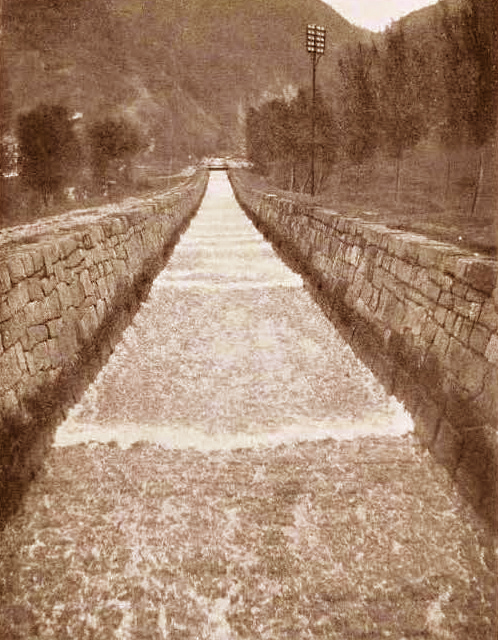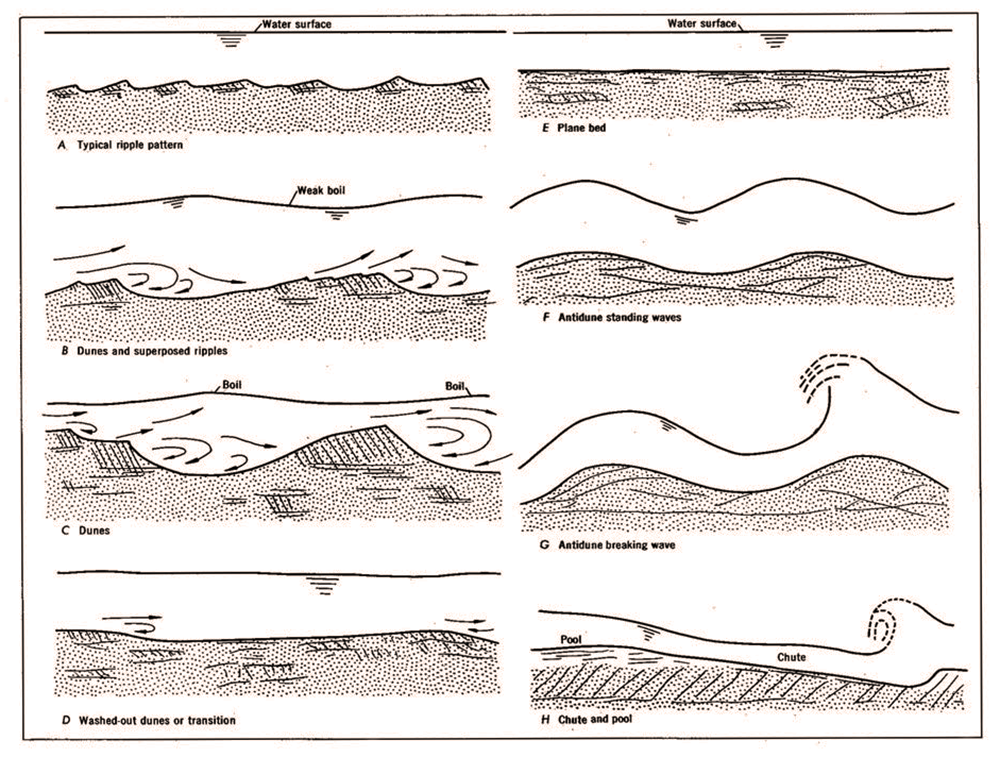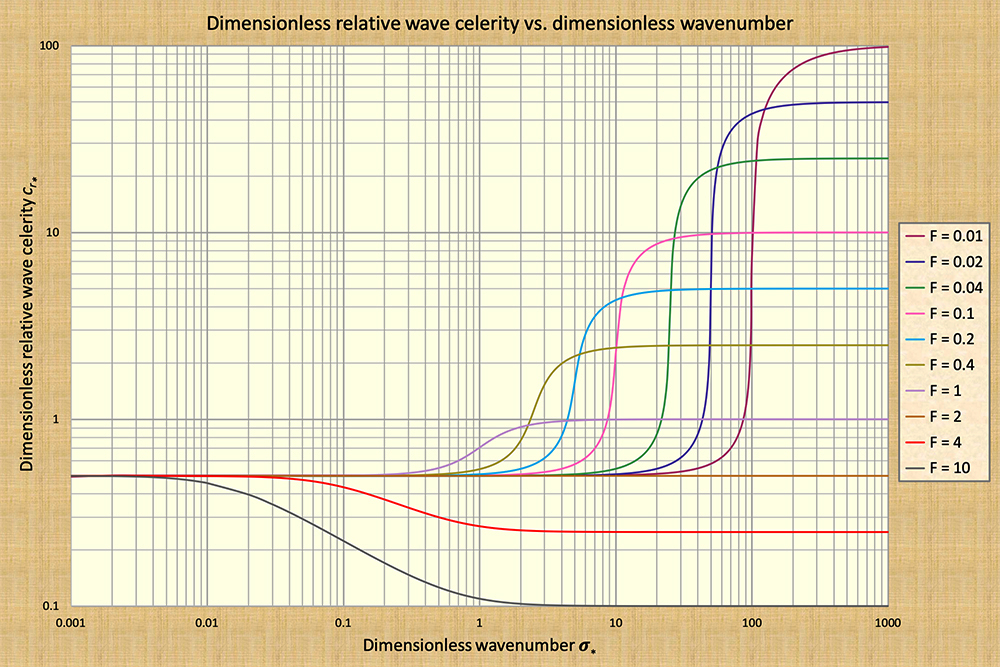Cornish (1907)
 Early fotograph of a train of roll waves in the Swiss Alps.

THE STATES OF FLOW

Victor M. Ponce

Professor Emeritus of Civil and Environmental Engineering

San Diego State University, San Diego, California

September 2, 2023

 ABSTRACT.  Four dimensionless numbers in open-channel flow are presented, explained and compared. Two of them are ratios of velocities, and the other two are ratios of diffusivities. All four numbers are defined in terms of veritable physical quantities, either velocities or diffusivities. Taken together, these numbers complete the description of the state of flow, for either steady flow (the first two numbers), or unsteady flow (the last two).

1.  INTRODUCTION

There are two characteristic properties in open-channel flow: (1) velocity; and (2) diffusivity. The velocity of a fluid parcel is the rate of change of its position in space, in a particular direction, with time. The units of velocity are L/T, in which L = length, and T = time. The expression u = 1 m/sec (one meter per second) describes the mathematical certainty that a chosen fluid parcel is moving along an established flow direction, or path, with a velocity u equal to 1 meter per second. In fluid mechanics, velocity relates to the process of convection of a fluid parcel; in hydrology, it relates to concentration, a concept related to time of concentration. In hydraulic and hydrologic modeling, velocity is described by a differential equation of first order.

Surface flow diffusivity ν is the first moment of the flow velocity. The units of diffusivity are (L/T)L, or its equivalent L2/T. The expression ν = 1 m2/sec, in relation to a given disturbance, describes the mathematical certainty that the disturbance is spreading at the rate controlled by the coefficient of diffusivity ν. In fluid mechanics, diffusivity relates to the process of diffusion; in hydrology, it relates to flood wave attenuation, or dissipation. In hydraulic and hydrologic modeling, diffusivity is described by a differential equation of second order (Table 1).

 Table 1.  Velocities and diffusivities in open-channel flow. Property Symbol Units Process Order Velocity u L/T Convection First Diffusivity ν L2/T Diffusion Second

These two fluid properties, velocity and diffusivity, characterize the flow up to second order. Several types of velocities and diffusivities may be construed, with their ratios constituting the dimensionless parameters referred to as "numbers." The latter encapsulate the properties of fluid flow, enhancing their understanding under both steady and unsteady conditions. This fact is reflected in the title of the present article: The states of flow, referring to the various states under which the flow may be described using these numbers. The remainder of this article endeavors to explains the numbers, clarifying their definition and scope.

2.  VELOCITIES IN OPEN-CHANNEL FLOW

There are three characteristic velocities in open-channel flow: (1) the mean velocity of steady flow u; (2) the relative celerity of kinematic waves v; and (3) the relative celerity of dynamic waves w. Celerity is the velocity of a wave (unsteady flow), as opposed to the velocity of steady flow (Ponce, 1991).

The celerity of a kinematic wave is: ck = β u, in which β = exponent of the rating, Q = αAβ, in which Q = discharge, A = flow area, and α = coefficient of the rating. Therefore, the relative celerity of a kinematic wave is:  v = ck - u,  i.e., the velocity of the kinematic wave relative to that of the flow (Ponce, 2014a).

The celerity of a dynamic wave, which has two components, is: cd = u ± (gD)1/2, in which g = gravitational acceleration, D = hydraulic depth, with D = A /T, and T = channel (stream) top width. Therefore, the relative celerity of a dynamic wave is:  w = cd - u = ± (gD)1/2,  i.e., the velocity of a dynamic wave relative to that of the flow (Ponce, 2014b).

The three velocities identified here encompass both steady (u) and unsteady flow (v and w), as well as short waves (dynamic, w), and long waves (kinematic, v). We note that these are the only flow velocities that are identifiable in the present context.

3.  DIFFUSIVITIES IN OPEN-CHANNEL FLOW

Three diffusivities are recognized in open-channel flow: (1) molecular diffusivity; (2) hydraulic diffusivity; and (3) spectral diffusivity. In fluid mechanics, the molecular diffusivity νm is commonly referred to as kinematic viscosity ν, a measure of the fluid's internal resistance to flow at the molecular level. In open-channel flow, the hydraulic diffusivity is expressed in terms of the bottom slope and bottom friction. In unsteady open-channel flow, the spectral diffusivity is defined in terms of the wavelength of the sinusoidal perturbation to the steady flow. These propositions are explained in Box A.

 Box A.  Diffusivities in open-channel flow. Newton's law of viscosity is: τ /ρ = ν (∂u/∂s), in which τ = shear stress, ρ = mass density of the fluid, ν = kinematic viscosity of the fluid, and (∂u/∂s) = velocity gradient in the direction s perpendicular to the direction of τ. τ /ρ = νm (∂u/∂s), in which νm = molecular diffusivity. The molecular diffusivity νm may be expressed as νm = u (Lm /2), in which Lm = (2νm /u)  is a characteristic molecular length (Chow, 1959). The hydraulic diffusivity νh is defined as νh = u (Lo /2), in which Lo = (do /So) is a characteristic hydraulic (reach) length, defined as the distance along the channel wherein the flow drops an elevation equal to its equilibrium depth (Hayami, 1951; Ponce and Simons, 1977). The spectral diffusivity νs is defined as νs = u (L /2), in which L = characteristic wavelength of the sinusoidal surface perturbation (Ponce, 1979). Note that all three diffusivities: (1) molecular, (2) hydraulic, and (3) spectral, are defined in terms of their respective characteristic lengths: (1) molecular length, (2) hydraulic (reach) length, and (3) spectral wavelength of the sinusoidal perturbation. Furthermore, note that the three diffusivities share a similar structure: A product of the convective velocity times one-half of a respective characteristic length.

4.  STEADY FLOW VELOCITY RATIO:  THE FROUDE NUMBER

The Froude number is the ratio of mean flow velocity u to relative celerity of dynamic waves w: F = u /w (Ponce, 2014b). It compares the mean flow velocity to the relative celerity of small (short) surface perturbations; accordingly, it classifies the flow into three types: (1) subcritical, for F < 1; (2) critical, for F = 1; and supercritical, for F > 1.

In open-channel hydraulics, the Froude number is useful in determining the direction of computation in the analysis of water surface profiles: Upstream for subcritical flow, and downstream for supercritical flow. In river mechanics, the Froude number is an indication of the limit between lower regime, F < 0.5, generally where ripples and dunes predominate in the streambed, and upper regime, F > 0.5, where plane bed and antidunes prevail (Simons and Richardson, 1966).

 [Click on top of figure to expand]Simons and Richardson (1966).
 Fig. 1   Forms of bed roughness in alluvial channels: (a) lower regime (left), and (b) upper regime (right).

Note the sharp division between subcritical and supercritical flow at F = 1. The latter constitutes a singular point, at which the direction of computation switches instantaneously between upstream and downstream. The existence of a singularity in the neighborhood of F = 1 may render the computation unstable; therefore, caution is recommended in the vicinity of critical flow. A history of the Froude number, including Froude's significant contributions to hydraulic engineering, has been presented by Ponce (2014b).

5.  STEADY FLOW DIFFUSIVITY RATIO:  THE REYNOLDS NUMBER

The Reynolds number is a ratio of viscosities, or diffusivities. The conventional Reynolds number, defined for an arbitrary cross-sectional shape, in terms of hydraulic radius Ro, is: R = (uo Ro) / νm (Ponce, 2014b). For a hydraulically wide channel: R = (uo do) / νm. For the purposes of this article, we define an alternative Reynolds number as an appropriate ratio of diffusivities, as follows: R' = νh/νm. Therefore: R' = R / (2 So) (see Table 2).

The Reynolds number R classifies the flow as being in one of the following regimes: (1) laminar, (2) transitional, or (3) turbulent. In open-channel flow, under steady flow conditions, laminar flow occurs for R ≤ 500; turbulent flow for R > 2000; and transitional flow in the intermediate range (500 < R ≤ 2000). The use of the Reynolds number is somewhat limited in open-channel flow applications, since the flow usually remains within the turbulent regime.

6.  UNSTEADY FLOW VELOCITY RATIO:  THE VEDERNIKOV NUMBER

The Vedernikov number is the ratio of the relative celerity of a kinematic wave v to the relative celerity of a dynamic wave w: V = v /w (Ponce, 2014b). It characterizes the following states of flow:

• V < 1:  Stable flow, for v < w,

• V = 1:  Neutrally stable flow, for v = w,

• V > 1:  Unstable flow, for v > w.

Under stable flow, the relative kinematic wave celerity v is smaller than the relative dynamic wave celerity w and, therefore, surface waves (perturbations) are able to attenuate (dissipate).

Under neutrally stable flow, the relative kinematic wave celerity v is equal to the relative dynamic wave celerity w and, therefore, surface waves neither attenuate nor amplify.

Under unstable flow, the relative kinematic wave celerity v is greater than the relative dynamic wave celerity w. Therefore, surface waves are subject to negative attenuation, i.e., amplification. In practice, the condition V > 1 leads to the development of roll waves, recognized as a train of waves that travel downstream, typically in artificial channels of steep slope (Fig. 2). The flow condition leading to the formation of roll waves may be explained in terms of the dynamic waves, which transport energy, overcoming the kinematic waves, which transport mass (Craya, 1952; Ponce and Choque Guzman, 2019).Fig. 2   A train of roll waves in a lateral canal, Cabana-Mañazo irrigation, Puno, Peru.

The theory of the Vedernikov number (Vedernikov, 1945; 1946), originally christened by Powell (1948) and later presented by Chow (1959) in Chapter 8 of his textbook, was clarified by Ponce (1991), who expressed the Vedernikov number in terms of relative kinematic and dynamic wave celerities. The subject of hydrodynamic stability for the control of roll waves in channelized rivers has been treated by Ponce and Choque Guzman (2019).

7.  UNSTEADY FLOW DIFFUSIVITY RATIO:  DIMENSIONLESS WAVENUMBER

The dimensionless wavenumber of Ponce and Simons (1977) is defined as follows: σ* = (2π /L)Lo. It may also be readily expressed as a ratio of diffusivities: σ* = (2π /L)Lo = 2π (νh /νs). The dimensionless wavenumber σ* classifies the unsteady flow being considered into four spectral ranges (Fig. 3):

1. Kinematic (to the extreme left),

2. Diffusion (left to left-of-center),

3. Mixed kinematic-dynamic (right-of-center to right), and

4. Dynamic (right to extreme right).

The precise domains of these spectral ranges have been determined by Ponce (2023):
• Kinematic flow: σ* < 0.001.

• Diffusion flow: 0.001 ≤ σ* < 0.17.

• Mixed kinematic-dynamic flow: 0.17 ≤ σ* < 1 to 100, depending on the Froude number (refer to Fig. 3).

• Dynamic flow: σ* ≥ 10 to 1000, depending on the Froude number (refer to Fig. 3).Ponce and Simons (1977)

Fig. 3   Dimensionless relative wave celerity cr* vs dimensionless wavenumber σ*.

The findings of Ponce and Simons (1977) help to elucidate the behavior of all possible wave types in unsteady open-channel flow. The latter include both "long" waves, ostensibly of a kinematic nature, on the far left side of Fig. 3, and "short" waves, of a dynamic nature, on the far right. Also included are the diffusion waves, of intermediate range and displaying properties that are shown to be quite practical, and the mixed kinematic-dynamic waves, for short mixed waves, in the middle-to-right range. These mixed waves are, for the most part, impractical due to their extremely strong dissipative tendencies (Ponce, 2023).

8.  SUMMARY

Four dimensionless numbers in open-channel flow are presented, explained and compared (Table 2). Two of them are ratios of velocities and the other two are ratios of diffusivities. All four numbers are defined in terms of veritable physical quantities, be it either velocities or diffusivities. Taken together, these numbers complete the description of the state of flow, for either steady flow (the first two numbers), or unsteady flow (the last two).

 Table 2.  Dimensionless numbers in open-channel flow. Dimensionless No. Symbol Ratio of Definition Ranges Alternate Froude F Velocities u / w (a) subcritical,(b) critical, (c) supercritical None Reynolds R' Diffusivities νh /νm (a) laminar,(b) transitional,(c) turbulent R Vedernikov V Velocities v / w (a) stable,(b) neutral,(c) unstable None Ponce-Simons σ* Diffusivities 2π (νh /νs) (a) kinematic,(b) mixed,(c) dynamic None

REFERENCES

Chow, V. T. 1959. Open-channel hydraulics. McGraw-Hill, Inc, New York, NY.

Cornish, V. 1907. Progressive waves in rivers. Journal of the Royal Geographical Society, Vol. 29, No. 1, January, 23-31.

Craya, A. 1952. The criterion for the possibility of roll wave formation. Gravity Waves, National Bureau of Standards Circular No. 521, National Bureau of Standards, Washington, D.C. 141-151.

Hayami, I. 1951. On the propagation of flood waves. Bulletin, Disaster Prevention Research Institute, No. 1, December, Extract.

Ponce, V. M. and D. B. Simons. 1977. Shallow wave propagation in open channel flow. Journal of Hydraulic Engineering ASCE, 103(12), 1461-1476.

Ponce, V. M. 1979. On the classification of open channel flow regimes. Proceedings, Fourth National Hydrotechnical Conference, Vancouver, British Columbia, Canada.

Ponce, V. M. 1991. New perspective on the Vedernikov number. Water Resources Research, Vol. 27, No. 7, 1777-1779, July.

Ponce, V. M. 2014. Fundamentals of Open-channel Hydraulics. Online text.

Ponce, V. M. and B. Choque Guzman. 2019. The control of roll waves in channelized rivers. Online article.

Ponce, V. M. 2023. When is the diffusion wave applicable? Online article.

Powell, R. W. 1948. Vedernikov's criterion for ultra-rapid flow. Transactions, American Geophysical Union, Vol. 29, No. 6, 882-886.

Simons, D. B. and E. V. Richardson. 1966. Resistance to flow in alluvial channels. Geological Survey Professional Paper 422-J, U.S. Government Printing Office, Washington, D.C.

Vedernikov, V. V. 1945. Conditions at the front of a translation wave disturbing a steady motion of a real fluid. Doklady Akademii Nauk USSR, 48(4), 239-242.

Vedernikov, V. V. 1946. Characteristic features of a liquid flow in an open channel. Doklady Akademmi Nauk USSR, 52(3), 207-210.

 230906